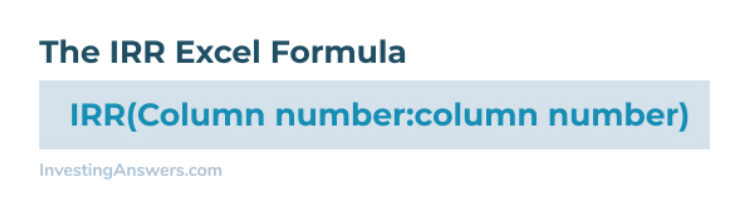Knowing how to calculate internal rate of return (IRR) is important for determining whether an investment is a good choice for your company. IRR is the discount rate that results in the investment’s net present value of zero. In other words, the IRR is a “break even” rate of return on the investment.

## How to Calculate IRR in Excel

Though it isn’t a straightforward calculation, Excel’s IRR formula makes it simpler to figure out your IRR for instant investment decisions.

### IRR Excel Formula

You can use the IRR function in Excel and you’ll need the following values:

• The initial investment (which would be negative cash flow)

• The cash flow value for each period

Input each figure into the same column and instruct Excel to compute the IRR by using the following IRR Excel formula:Input this number into the cell directly below the column with your cash flow numbers.

## IRR Calculator in Excel Example

Here’s a simple example of how to find IRR in Excel using the following figures:

• Initial investment: \$50,000

• Subsequent cash flows: \$20,000 each year for 5 years; \$40,000 in year 6.

## How to Find IRR on a Financial Calculator

If you don’t use Excel, you can still calculate IRR using a financial calculator (such as the Texas Instrument BA II Plus). You’ll need the same figures you’d use to find IRR in Excel: the initial investment and subsequent cash flows.

Using a financial calculator, enter the following:

• Press the CF (Cash Flow) button to start the Cash Flow register.

• Enter the initial investment (negative number).

• Hit enter.

• Hit the down arrow to move to CF1 or your first year’s cash flow.

• Enter the amount for year 1.

• Hit the down arrow twice to enter year 2’s cash flow.

• Repeat the process until you’ve entered each year of projected cash flow.

• Press the IRR key.

• Press the CPT key for your IRR.

## Calculating IRR with a Financial Calculator Example

Here’s an example of how to find IRR with a financial calculator using the following figures:

• Initial investment: \$150,000

• Subsequent cash flows: \$50,000 per year for 5 years.

### Step 1: Press the Cash Flow (CF) Button

This starts the Cash Flow Register when you enter your initial investment. Because cash is paid out, it’s a negative number. In this example, enter -\$150,000 and hit enter.

### Step 2: Press the Down Arrow Once

The calculator should show CF1. This is where you enter the first year’s cash flow. For this example, enter \$50,000.

### Step 3: Press the Down Arrow Twice

The calculator should show CF2. This is where you'll enter the second year’s cash flow. In this example, enter \$50,000.

### Step 4: Repeat

Press the down arrow two more times and repeat Step 3 until you’ve entered all five years of \$50,000 cash flow.

### Step 5: Press the IRR Key

Press the IRR key to start the IRR calculation, followed by the CPT key. This should provide the IRR calculation for your investment.